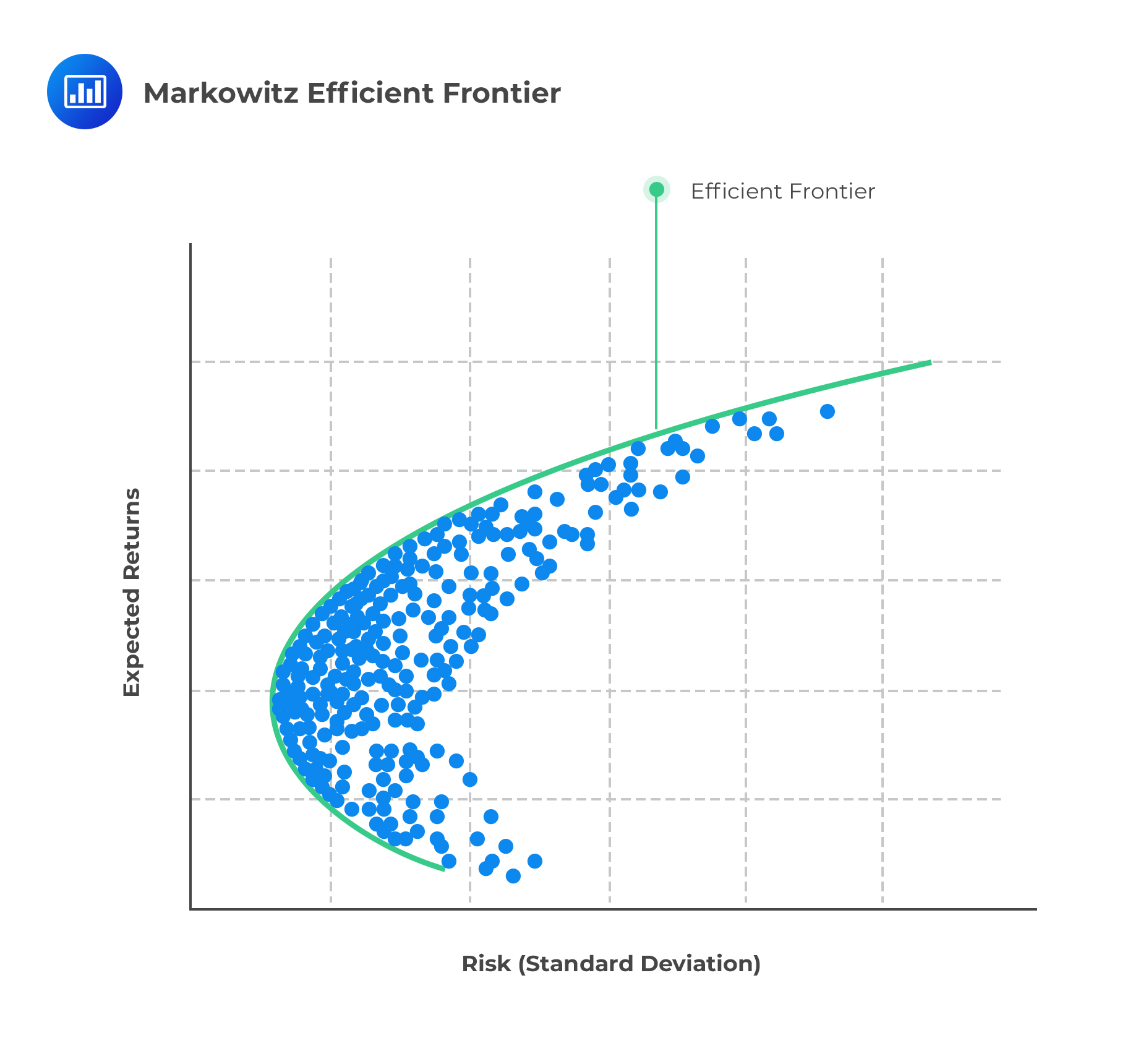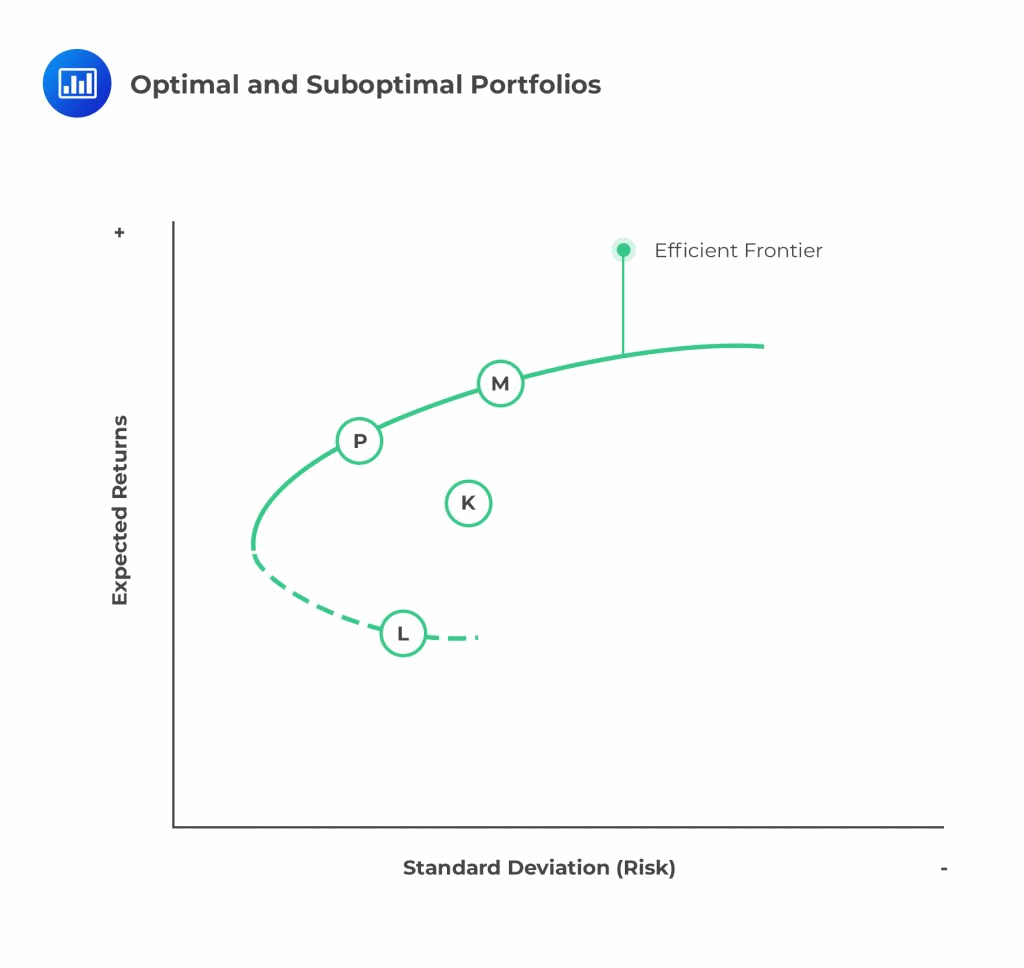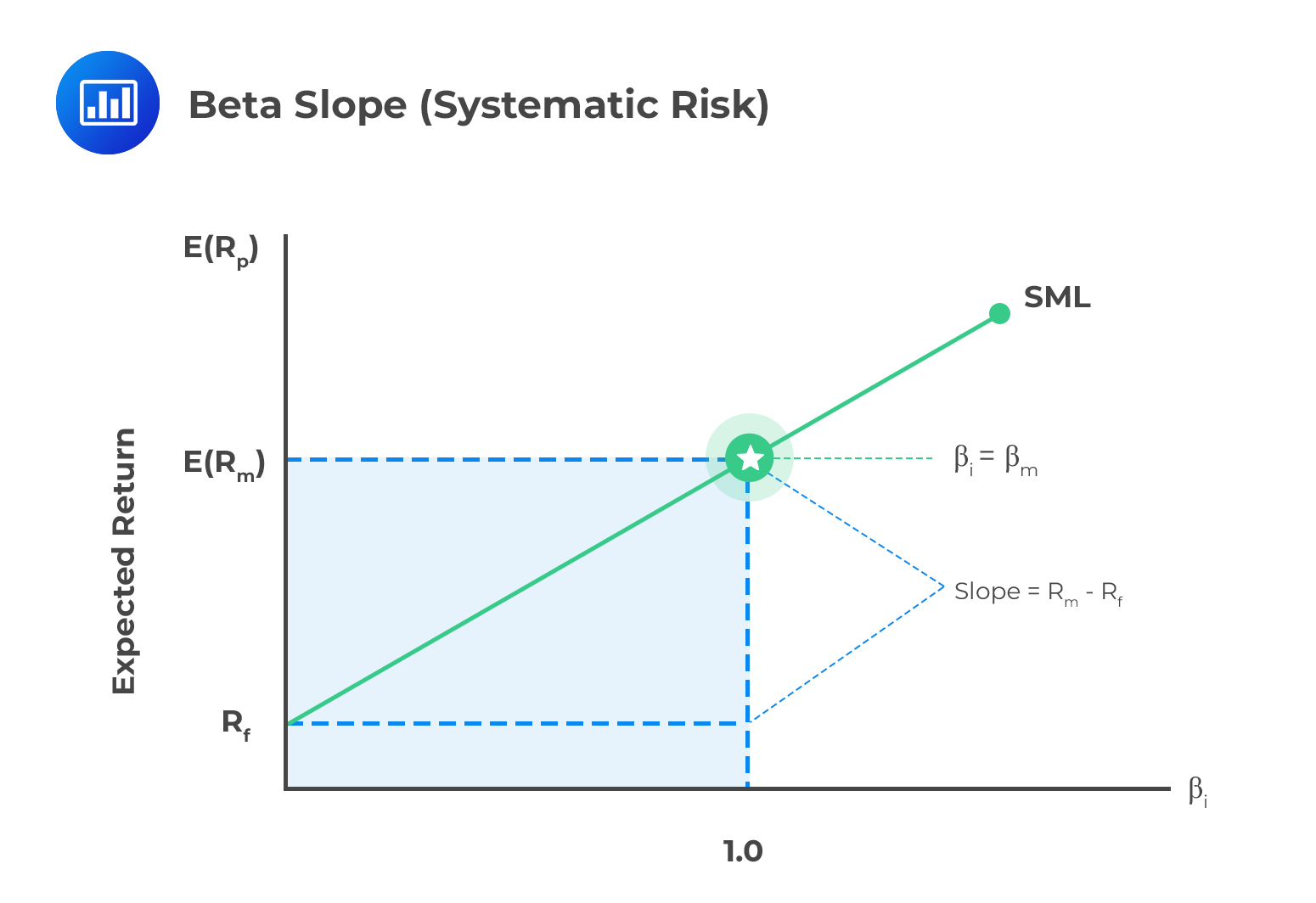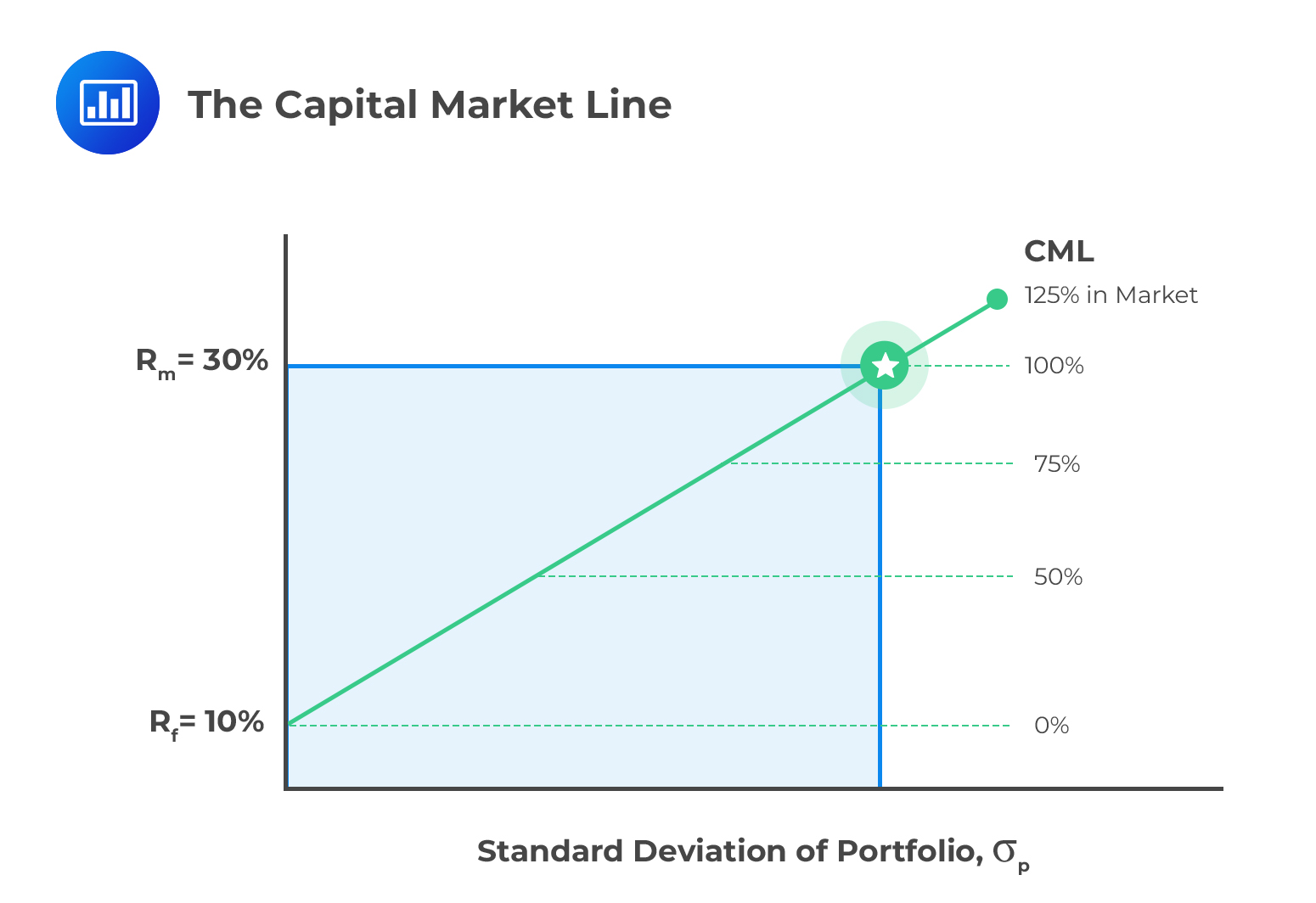Limited Time Offer: Save 10% on all 2022 Premium Study Packages with promo code: BLOG10# Modern Portfolio Theory (MPT) and the Capital Asset Pricing Model (CAPM)

After completing this reading, you should be able to:

• Explain modern portfolio theory and interpret the Markowitz efficient frontier.
• Understand the derivation and components of the CAPM.
• Describe the assumptions underlying the CAPM.
• Interpret and compare the capital market line and the security market line.
• Apply the CAPM in calculating the expected return on an asset.
• Interpret beta and calculate the beta of a single asset or portfolio.
• Calculate, compare, and interpret the following performance measures: the Sharpe performance index, the Treynor performance index, the Jensen performance index, the tracking error, information ratio, and the Sortino ratio.

Exam tip: Be sure to understand the calculations behind the CAPM because there is a strong likelihood you will be getting mathematical questions on this in your FRM part 1 exam.

## Modern Portfolio Theory

Modern portfolio theory is attributed to Harry Markowitz, who postulated that a rational investor should evaluate the potential portfolio allocations based on means and variances of the expected return distributions.

## Assumptions of the Modern Portfolio Theory

I. The capital markets are perfect. Perfect market implies that:

• There are no transaction costs and taxes.
• All market participants can access available information without any cost.
• There is perfect competition in the market.

II. The returns from the portfolios are normally distributed. This allows the characteristics of the returns to be stated in terms of the mean and the variance.

Markowitz suggested that the size of investment made by an institution should be based on the contribution of the assets to the entire portfolio’s return (in terms of mean and return). The assets’ performance is not evaluated independently but rather with the performance of other assets.

Portfolio diversification is one method of decreasing the risk exposure to each asset. Thus, investors must be compensated for accepting the risk in each asset.   Diversification enables the maximization of the level of returns at any provided level of risk.

## Markowitz Efficient Frontier

The Markowitz Efficient Frontier (or only efficient frontier) is a curved solid curve with a plot of the optimal returns for each level of risk. Each point on the curve represents the maximum level of portfolio return for a given level of risk.Now, consider the following efficient frontier.Portfolio P gives the best return for the same level of risk. On the other hand, portfolio K is termed as suboptimal since there are other portfolios (located on the vertical distance between the portfolio K and the efficient frontiers) that can offer better returns for the same level of risk, namely portfolio M.

Portfolio M is referred to as the market portfolio. The market portfolio assumes that the market attains the equilibrium and appropriately includes all the risky assets in the economy weighted by their respective market values.

Portfolio P is also an efficient portfolio but representing a lower level of risk than portfolio M.

From the efficient frontier, it is easy to see that the expected return is increased by increasing the portfolio’s risk level and vice versa. The dotted line represents the most inefficient portfolios; in this case, portfolio L. Inefficient portfolios imply that the investor achieves the lowest level of return given any level of risk.

## The Capital Asset Pricing Model (CAPM)

The Capital Asset Pricing Model, derived by Sharpe, Lintner, and Mossin, stipulates assumptions regarding the market and how investors behave to enable the creation of an equilibrium model of prices in the whole market. CAPM explains that the market equilibrium is attained when all investors hold portfolios whose constituents are a combination of riskless assets and the market portfolio.

## Assumptions Underlying the CAPM

Assumptions of the CAPM model include:

• There are no transaction costs
• There are no taxes
• Assets are infinitely divisible
• Unlimited short-selling is permissible
• All assets are marketable/liquid
• Investors are price takers whose individual buy and sell transactions do not affect the price
• Investors’ utility functions are based solely on expected portfolio return and risk
• The only concern among investors are risk and return over a single period, and the single period is the same for all investors.

Under these assumptions, the expected rate of return over a given holding time is given by:

$$E\left( { R }_{ i } \right) ={ R }_{ f }+{\beta}_i \left( { R }_{ m }-{ R }_{ f } \right)$$

Where

$$E(R_i )$$: the expected return of asset i over the holding period

$$R_f$$: rate of return on the risk-free asset

$$R_m$$: Expected market return over the holding period

$$β_i$$: the Beta factor of the asset i

Note that $$\left( { R }_{ m }-{ R }_{ f } \right)$$ is the expected return per unit risk (beta) and $${\beta}_i \left( { R }_{ m }-{ R }_{ f } \right)$$ is the expected return above the risk-free rate of return.

## Interpreting Beta

Beta is a measure of the systematic risk associated with a particular stock, asset, or portfolio. Systematic risk is the portion of risk that cannot be eliminated by any amount of diversification.A value of beta above 1 indicates a stock/asset/portfolio that has, historically, amplified the return of the whole market (positive or negative). A beta close to zero would indicate a stock/asset/portfolio that provides a more stable return than the market as a whole. A negative beta would signify a stock/asset/portfolio whose performance is counter-cyclical, i.e., offsets the overall market experience.

For company $$i$$:

$${\beta}_{i}=\frac{Cov(i,m)}{{\sigma}_{m}^{2}}=\frac {{\sigma}_{im}}{ {\sigma}_{m}^{2}}$$

Where $${ \sigma }_{ m }$$ is the variance of the market index and $${ \sigma }_{ im }$$ the covariance between the individual stock’s/asset’s return and that of the market.

Alternatively, by using the fact that:

$$Corr(i,m)={\rho}_{im}=\frac{Cov(i,m)}{{\sigma }_{ i }{\sigma}_{ m }}=\frac{{\sigma}_{im}}{{ \sigma }_{ i }{ \sigma }_{ m }}$$

$$\Rightarrow { \sigma }_{im}={\rho}_{im}.{ \sigma }_{ i }{ \sigma }_{ m }$$

We can write:

$${ \beta }_{ i }={ \rho }_{ im }\frac { { \sigma }_{ i } }{ { \sigma }_{ m } }$$

Where

$$ρ_{im}$$: correlation coefficient between returns of asset i and that of the market portfolio.

$$σ_i$$: standard deviation of asset i

$$σ_m$$: standard deviation of the market portfolio

Therefore, we can write:

$$E(R_i) = R_f +{\sigma}_{i} {\rho}_{im} \left(\frac{R_m – R_f}{{\sigma}_{m}}\right)$$

This is the equation of the security market line (SML). The equation implies that the expected return on any asset is equivalent to a risk-free rate of return plus the premium. The SML implies that the expected return on any asset can be expressed as the linear function of assets covariance with the whole market.

## Derivation of CAPM

The derivation of CAPM involves three major steps:

1. Recognize that investors are only compensated for bearing systematic risk, not specific risks that can easily be diversified away. Note that, beta is an appropriate measure of systematic risk.
2. Suppose we recognize that portfolio expected return is a weighted average of individual expected returns and portfolio beta is a weighted average of the individual betas. In that case, we can show that portfolio return is a linear function of portfolio beta. And because arbitrage prevents mispricing of assets relative to systematic risk, then an individual asset’s expected return is a linear function of its beta.
3. We can then use the risk-free asset and the market portfolio to solve for the intercept and slope of the CAPM.

$$E\left( { R }_{ i } \right) ={ R }_{ f }+\beta \left( { R }_{ m }-{ R }_{ f } \right)$$

## The Capital Market Line

The capital market line expresses the expected return of a portfolio as a linear function of the risk- free rate, the portfolio’s standard deviation, and the market portfolio’s return and standard deviation.

$$E\left( { R }_{ C } \right) ={ R }_{ f }+\left[ \frac { E\left( { R }_{ m } \right) -{ R }_{ f } }{ { \sigma }_{ m } } \right] { \sigma }_{ C }$$

Where

$$E(R_C)$$ is the expected return of any portfolio on the efficient frontier

$$σ_C$$ is the standard deviation of the return on portfolio C

$$E(R_m )$$   is  the expected return on the market portfolio

$$σ_m$$ is the standard deviation of the return on the market portfolio

$$R_f$$ is the risk-free rate of return.The capital market return implies that the return on any portfolio is a linear function of its standard deviation. The variable $$\left[ \frac { E\left( { R }_{ m } \right) -{ R }_{ f } }{ { \sigma }_{ m } } \right]$$ is termed as the market price of risk or the risk premium.

## The Sharpe Performance Index (SPI)

The Sharpe ratio is equal to the risk premium divided by the standard deviation:

$$SPI=\frac { E\left( { R }_{ p } \right) -{ R }_{ f } }{ \sigma \left( { R }_{ P } \right) }$$

Where:

$$E\left( { R }_{ p } \right)$$ = portfolio’s expected return

$${ R }_{ f }$$ = risk-free rate

$$\sigma \left( { R }_{ P } \right)$$ = standard deviation of returns of the portfolio

The Sharpe ratio, or reward-to-variability ratio, is the slope of the capital allocation line (CAL). The greater the slope (higher number), the better the asset. Note that the risk being used is the total risk of the portfolio, not its systematic risk, which is a limitation of the measure. The portfolio with the highest Sharpe ratio has the best performance, but the Sharpe ratio by itself is not informative. In order to rank portfolios, the Sharpe ratio for each portfolio must be computed.

A further limitation occurs when the numerators are negative. In this instance, the Sharpe ratio will be less negative for a riskier portfolio resulting in incorrect rankings.

## The Treynor Performance Index (TPI)

The Treynor measure (1965) of a portfolio is explained by the expression below.

$$TPI=\frac { E\left( { R }_{ p } \right) -{ R }_{ f } }{ { \beta }_{ p } }$$

Where:

$$E\left( { R }_{ p } \right)$$ = portfolio’s expected return

$${ R }_{ f }$$ = risk-free rate

$${ \beta }_{ p }$$ = beta of the portfolio

Treynor measures the risk premium per unit risk (Beta). As with the Sharpe ratio, the Treynor ratio requires positive numerators to give meaningful comparative results and the Treynor ratio does not work for negative beta assets. Also, while both the Sharpe and Treynor ratios can rank portfolios, they do not provide information on whether the portfolios are better than the market portfolio or information about the degree of superiority of a higher ratio portfolio over a lower ratio portfolio.

## The Jensen Performance Index (JPI)

Jensen’s alpha (Jensen, 1968) is described as an asset’s excess return over and above the return predicted by CAPM.

$$\text{ Jensen’s measure of a portfolio }={ \alpha }_{ p }=E\left( { R }_{ p } \right) -\left[ { R }_{ f }+{ \beta }_{ p }\left( E\left( { R }_{ m } \right) -{ R }_{ f } \right) \right]$$

Jensen’s alpha is based on systematic risk. The daily returns of the portfolio are regressed against the daily market returns to compute a measure of this systematic risk in the same manner as the CAPM. The difference between the actual return of the portfolio and the calculated or modeled risk-adjusted return is a measure of performance relative to the market.

If αp is positive, the portfolio has outperformed the market, whereas a negative value indicates underperformance. The values of alpha can be used to rank portfolios or the managers of those portfolios, with the alpha being a representation of the maximum an investor should pay for the active management of that portfolio.

### Relationships Between Treynor and Jensen Performance Indices

Denote the JPI by $${ \alpha }_{ p }$$ which is the y-intercept of the regression equation:

$$E\left(R_p\right)-R_f ={ \alpha }_{ p }+{ \beta }_{ p }\left(E\left(R_m\right)-R_f\right)$$

Divide the equation above by $${ \beta }_{ p }$$ to get:

$$\frac{E\left(R_p\right)-R_f}{{ \beta }_{ p }}=\frac{{ \alpha }_{ p }}{{ \beta }_{ p }} +\left(E\left(R_m\right)-R_f\right)$$

The left-hand side of the equation above is just TPI. So,

$$\text{Treynor Performance Index}=TPI=\frac{{ \alpha }_{ p }}{{ \beta }_{ p }} +\left(E\left(R_m\right)-R_f\right)$$

For a greater performance, $$TPI>E\left(R_m\right)-R_f$$  and thus  $$\frac{α_p}{β_p} >0$$. Also, $$β_p>0$$ for almost all assets and thus it must be true that $$α_p>0$$. From these results is evident to say that if a superior performance is demonstrated by TPI, then is also the case for JPI and vice versa.

## The Tracking Error (TE)

Tracking error measures the difference between a portfolio’s return and that of a benchmark level, which was meant to be surpassed by the tracking error. We need to calculate the quantity:

$$TE= \left( { R }_{ P }-{ R }_{ B } \right)$$

Where

$$R_P$$: the return of the portfolio is considered

$$R_B$$: Return on the benchmark portfolio

Another way of calculating the TE is to calculate the standard deviation of the difference in the portfolio and the benchmark return:

$$TE=\sqrt{\frac{\sum{(R_P-R_B)^2}}{N-1}}$$

Where N is the number of periods under consideration.

## The Information Ratio (IR)

The information ratio is similar to SPI, only that it is the active return relative to the benchmark portfolio divided by the standard deviation of the active return $$\left(R_P-R_B\right)$$:

$$IR=\frac{E(R_P-R_B)}{\sqrt{Var (R_P-R_B)}}$$

Where:

$${ R }_{ P }$$=portfolio return and

$${ R }_{ B }$$=benchmark return

IR can be used in place of TE

## The Sortino Ratio (SR)

The Sortino ratio is much like the Sharpe ratio, but there are two glaring differences:

• The risk-free rate is replaced with a minimum acceptable return, denoted as $${ R }_{ min }$$
• The standard deviation is replaced by a semi-standard deviation, which measures the variability of only those returns that fall below the minimum acceptable performance.

The measure of risk is the square root of the mean squared deviation from T of those observations in period t where $$T > R_{Pt}$$, else zero.

$$SR=\frac{(E(R_P )-T}{\sqrt{\frac{1}{N}\sum_{t=1}^{N}{min(0,R_{Pt} -T)^2}}}$$

Where T is the target or required rate of return (which can be a risk-free rate or any other rate) for an investment decision. It is also termed as the minimum acceptable rate of return (MAR).

## Question 1

Given the following information for a security:

• Rf = 5%
• Std. dev. of security = 40%
• Security correlation with market = 0.80
• Std. dev. of market = 20%
• Rm = 10%

What is the expeceted return for the security?

A. 16%

B. 13%

C. 21%

D. 24%

The correct answer is B.

First, find Beta;

• Beta = (0.80 x 0.40)/0.20 = 1.6

Next, use the CAPM model to find the expected return;

$$E(R_i)=R_f+β_i(R_m-R_f)$$

• E(Ri) = 5% + 1.6(10% – 5%) = 13%

## Question 2

Given the following information:

• Risk-free asset: Rf = 3%
• Market portfolio: E(Rp) = 10%
• Standard deviation: σ = 26%

What is the expected return from a portfolio which has 130% weight invested in the risky asset?

A. 14.8%

B. 8.4%

C. 12.1%

D. 13%

The correct answer is C.

Here, we’re borrowing 30% in the risk-free asset and investing the proceeds plus the whole portfolio in the market portfolio.

Return with -30% in the risk-free asset and 130% in the risky asset:

• E(Ri) = -0.3 × 3% + 1.3 × 10% = 12.1%

## Question 3

A portfolio has an expected return of 18% and a volatility of 10%. If the risk-free rate of interest is 4%, then what is the Sharpe ratio of the portfolio?

A. 0.14

B. 0.18

C. 1.8

D. 1.4

The correct answer is D.

$${ S }_{ P }=\frac { E\left( { R }_{ p } \right) -{ R }_{ f } }{ \sigma \left( { R }_{ P } \right) }$$

Sharpe Ratio of the portfolio = (0.18-0.04)/0.10 = 1.4

## Question 4

Your portfolio had a value of EUR 1,000,000 at the start and EUR 1,150,000 at the end of the year. Over the same period, the benchmark index has had a return of 4%. If the tracking error is 11%, then what is the information ratio?

A. 1

B. 0.11

C. 0.733

D. 1.36

The correct answer is A.

The return of the portfolio is (1,150,000 – 1,000,000) / 1,000,000 = 0.15 or 15%

$$IR=\frac { E\left( { R }_{ P } \right) -E\left( { R }_{ B } \right) }{ \text{Tracking error} }$$

= (15% – 4%) / 11% = 1

Shop CFA® Exam Prep

Offered by AnalystPrepLevel I
Level II
Level III
All Three Levels
Featured Shop FRM® Exam PrepFRM Part I
FRM Part II
FRM Part I & Part II
Learn with Us

Subscribe to our newsletter and keep up with the latest and greatest tips for success
Shop Actuarial Exams PrepExam P (Probability)
Exam FM (Financial Mathematics)
Exams P & FM
Shop GMAT® Exam PrepComplete CourseDaniel Glyn
2021-03-24
I have finished my FRM1 thanks to AnalystPrep. And now using AnalystPrep for my FRM2 preparation. Professor Forjan is brilliant. He gives such good explanations and analogies. And more than anything makes learning fun. A big thank you to Analystprep and Professor Forjan. 5 stars all the way!michael walshe
2021-03-18
Professor James' videos are excellent for understanding the underlying theories behind financial engineering / financial analysis. The AnalystPrep videos were better than any of the others that I searched through on YouTube for providing a clear explanation of some concepts, such as Portfolio theory, CAPM, and Arbitrage Pricing theory. Watching these cleared up many of the unclarities I had in my head. Highly recommended.Nyka Smith
2021-02-18
Every concept is very well explained by Nilay Arun. kudos to you man!2021-02-13